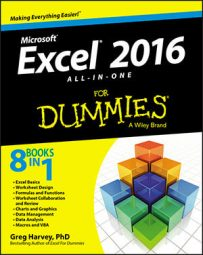##### Excel 2016 All-in-One For DummiesThe IS information functions in Excel 2016 (as in ISBLANK, ISERR, and so on) are a large group of functions that perform essentially the same task. They evaluate a value or cell reference and return the logical TRUE or FALSE, depending on whether the value is or isn't the type for which the IS function tests. For example, if you use the ISBLANK function to test the contents of cell A1 as in

`=ISBLANK(A1)`

Excel returns TRUE to the cell containing the formula when A1 is empty and FALSE when it's occupied by any type of entry.

Excel offers ten built-in IS information functions:

• ISBLANK(value) to evaluate whether the value or cell reference is empty

• ISERR(value) to evaluate whether the value or cell reference contains an Error value (except for #N/A)

• ISERROR(value) to evaluate whether the value or cell reference contains an Error value (including #N/A)

• ISLOGICAL(value) to evaluate whether the value or cell reference contains the logical TRUE or FALSE value

• ISNA(value) to evaluate whether the value or cell reference contains the special #N/A Error value

• ISNONTEXT(value) to evaluate whether the value or cell reference contains any type of entry other than text

• ISNUMBER(value) to evaluate whether the value or cell reference contains a number

• ISODD(number) to evaluate whether the value in the referenced cell is odd (TRUE) or even (FALSE)

• ISREF(value) to evaluate whether the value or cell reference is itself a cell reference

• ISTEXT(value) to evaluate whether the value or cell reference contains a text entry

In addition to these ten IS functions, Excel adds two more, ISEVEN and ISODD, when you activate the Analysis ToolPak add-in. The ISEVEN function evaluates whether the number or reference to a cell containing a number is even, whereas the ISODD function evaluates whether it is odd.